Determinants and Numerical Approximations to Zero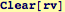Start by building a routine to make vectors containing six random numbers on the interval {-1,1}: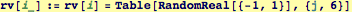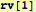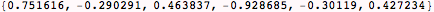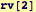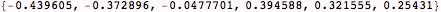Now use rv to make a 6 x 6 matrix, then find its determinant: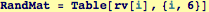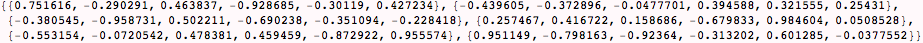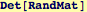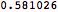Switching two rows changes the sign but not the magnitude of the determinant: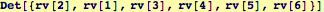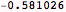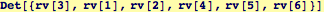Multiply one row by a constant and calculate determinant: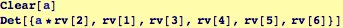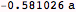Multiply two rows by a constant and calculate determinant: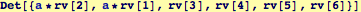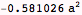Multiply all rows by a constant and calculate determinant: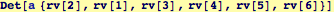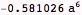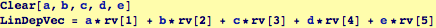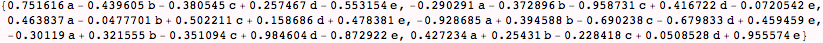Example of numerical precision: if one row of a 6 x 6 matrix is a linear combination of the other five rows, its determinant should evaluate to zero…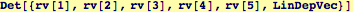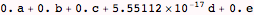However, numerical precision does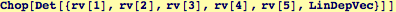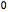Created by Wolfram Mathematica 6.0  (06 September 2007)